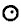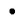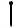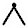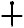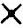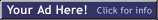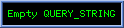SpaceFlights.us: Radius Calc
Posted in: News | by Brian Taylors | 2013 Oct 29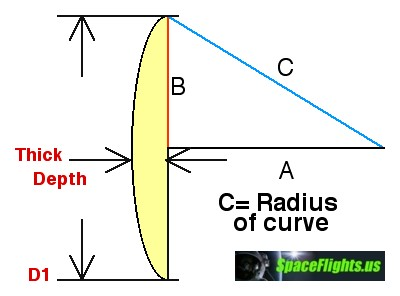Radius Calc
This diagram illustrates how to use a right angle triangle to compute the radius of a curve. It is part of a speadsheet data base I developed to calculate the focal point of a parabolic curve by taking it's diameter and how deep or thick the curve was.

It's application goes far beyond designing mirrors for telescopes. It can be used to compute the radius of train tracks or crop circles.
 Formula: C = ((Thick^2) + (B^2)) / (Thick x 2)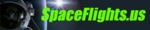Copyright 2019 by SpaceFlights.us